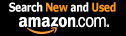CTK Exchange Front Page Movie shortcuts Personal info Awards Terms of use Privacy Policy Cut The Knot! MSET99 Talk Games & Puzzles Arithmetic/Algebra Geometry Probability Eye Opener Analog Gadgets Inventor's Paradox Did you know?... Proofs Math as Language Things Impossible My Logo Math Poll Other Math sit's Guest book News sit's Recommend this site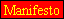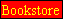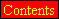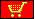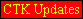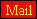CTK Exchange

 Subject: "Golden Number linked to 666" Previous Topic | Next Topic
 ConferencesThe CTK ExchangeThis and thatTopic #606Printer-friendly copyEmail this topic to a friend Reading Topic #606
Tit'sguest
Apr-30-05, 06:48 AM (EST)

 Golden Number linked to 666Formal Proof neededHello,While working on my websit's on 666 myth ( https://www.666myth.co.nr/ French= https://www.666mythe.co.nr/ ) and on Isomorphous Triplets ( https://www.chez.com/cosmos2000/Numbers/IsomorphousTriplets.html ) I have found recently direct links between the Beast Number 666 and the famous Golden Number Phi, well-known by Pythagoras, Leonardo da Vinci ... and in Sacred Geometry. This unexpected and incredible relation 666 versus Φ may constitute a way for the Rehabilitation of 666 !!! ...First we have this curious relations:666 = 7^3 pi (Phi – 1) = 7^3 pi phi or 666 = (6/5) 7^3 Phiwith pi = 3.141593 Phi = 1.618034 phi = Phi -1 = 0.618034 7^3 = cubic of 7Besides, after computing some sinus and cosines https://www.1728.com/trigcalc.htm >, expressed in Degrees and absolute values, we obtain: Phi /2 = sin 666ş = cos 324ş = cos 216ş = cos 144ş = cos 36ş = 0.80901699...Phi = 2sin 666ş = 2cos 324ş = 2cos 216ş = 2cos 144ş = 2cos 36ş = 1,61803399...Phi = sin 666ş + cos 216ş = sin 666ş + cos (6x6x6)şPhi = cos 144ş + cos 36ş = cos <(6+6) x (6+6)>ş + cos (6 x 6)şPhi = sin 666ş + cos 144ş = sin 666ş + cos <(6+6) x (6+6)>şBut, I am wondering if there could exist formal mathematical proofs for this 666 and Golden Number links.Thanks for advice and help.Titus (cosmos2000 (@) iquebec.com)

10scott10
Member since May-25-05
May-25-05, 10:15 PM (EST)1. "RE: Golden Number linked to 666"
In response to message #0

 the # of the beast is not 666, but 616. it was in the news recently

Corwin
Member since Jun-4-05
Jun-04-05, 08:21 PM (EST)2. "RE: Golden Number linked to 666"
In response to message #0

 https://www.cut-the-knot.org/htdocs/dcforum/DCForumID4/606.shtmlThere will always be a use for large prime numbers, eg 111, whenexpressing approximations to irrational numbers. There are 11*6 feetin a chain, 13 weeks in a quarter, and 2*11/7 = 3.1428 = Pi + 0.0013.666 - 720 = 54 = 270/5 deg = 3 Pi / 10 radians pi/5 is related to the golden ratio616 - 630 = 14 is not likely to be related to the golden ratio 5**1/2 = 2.2360679774997896964091736687313 (5/7)**1/2 = 0.84515425472851657750961832736595 (5/7)**1/3 = 0.89390353509656765128791642511372 1/ Phi = Phi -1 Phi**2 - Phi -1 = 0 2*Phi = 1 +-(1+4)**0.5 = 1 +- (5)**0.5 = 2* 1.6180339887498948482045868343656Phi and pentagonal symmetry are discussed in depth at https://www.mcs.surrey.ac.uk/Personal/r.Knott/Fibonacci/phi2DGeomTrig.html#trigmore 1/(5)**1/2 - 0.44721359549995793928183473374626 pentagonal symmetry pi/5 = 36deg sin36 = 0.58778525229247312916870595463907 cos36 = 0.80901699437494742410229341718282 = Phi /2 = (1+(5)**0.5)/4 tan36 = 0.72654252800536088589546675748062 = (5-2*5**0.5)**0.5 cos72 = ((5**0.5-1)**0.5/2 Thus in pentagonal symmetry the is a correspondence or relationship of Phi, 5**0.5 and Pi/5 . Why or how can this be derived? 36-54 triangle hypotenuse = 4 side opposite 36 = 2 Phi = 2.35114100 = 2*1.17557050 side opposite 54 = = 3.236067977I have observed the phenomenon when I calculated the dodecahedral andicosahedral angles. Consider a pentagonal jello mold with each ifthe five sides a cylinder centered at the center of the pentagon basewith unit vectors going from the center of the pentagon and scribingan arc to the vertical. At some angle the unit vector points areunity apart; this defines the dodecahedral symmetry. The sides ofthe base are then 2*sin(Pi/5) = 1.176 . If the five unit vectors areraised by an angle alphathen the sides of the elivated pentagon are2*cos(alpha)*sin(Pi/5) = 1 . dodecahedral, icosahedral symmetry In calculating the unit vectors to the vertices I observed Phi and/or the square root of 5 cropping up. cos(pi - dihedral) = 1/5**0.5We have levels of complexity Pi/2 2**0.5, Pythagoras, square root Pi/6 3**0.5 Pi/5 Phi, golden, quadratic equation root Pi/7 cubic equation root ...My discussion of possible and impossible things is at https://www.issi1.com/corwin/possible.txtcc:golden numerology cosmos2000@iquebec.com www.666myth.co.nr/ cosmos2000@iquebec.com?subject=666 Bivalent Myth www.chez.com/cosmos2000/Numbers/IsomorphousTriplets.htmlwww.liftbar.comCorwinguest
Jul-07-05, 10:36 PM (EST)

3. "RE: Golden Number linked to 666"
In response to message #2

 Wm.C. Corwin.info, Ph.D.,P.E. www.ConcurrentInverse.com billc@issi1.com (c) copyright 2005 Wm.C.CorwinTITLE: Proof of simple exact formulas for dimensions of the dodecahedron and icosahedron.mathworld@wolfram.com EricWWeisstein mathworld.wolfram.com/TrigonometricAdditionFormulas.htmlefr@st-andrews.ac.uk www-groups.dcs.st-andrews.ac.uk/~history/HistTopics/Golden_ratio.htmlThe proof of the exact formulas in closed form for the dodecahedronand icosahedron is at https://www.issi1.com/corwin/calculator/proof.txt .I have not yet read the formulas or their derivation elsewhere butGeorge Hart has informed me that they have been published. I don'tthink the derivation is on the internet so I am putting it there soreferences and links to them can be made. Interest in proofsof exact formulas for pentagonal symmetry was expressed athttps://www.cut-the-knot.org/htdocs/dcforum/DCForumID4/606.shtml .I have used the numerical results to make ray tracing applications at.RESULTS: dodecahedron face normal angle arccos(1/5**0.5) 63.4349488 deg edge length (50 - 22*5**0.5)**0.5 = 0.89805595 inscribed radius 1 center of edge radius ((5 - 5**0.5)/2)**0.5 = 1.17557 superscribed radius 3**0.5 * (5 - 2*5**0.5)**0.5 = 1.25840857 pentagon height (5/2)**0.5 * ( 3 - 5**0.5)**0.5 = 1.381966 surface area 30 * 2**0.5 * (65 - 29*5**0.5)**0.5 volume 10 * 2**0.5 * (65 - 29*5**0.5)**0.5 = 5.55029 4pi/3 = 4.1887 icosahedron face normal angle arccos(5**0.5/3) edge length 6**0.5 * (7 - 3*5**0.5)**0.5 inscribed radius 1 center of edge radius (3/2)**0.5 * (3 - 5**0.5)**0.5 superscribed radius 3**0.5 * (5 - 2*5**0.5)**0.5 triangle heigth 3/2**0.5 * (7 - 3*5**0.5)**0.5 surface area 30 * 3**0.5 * (7 - 3*5**0.5) volume 10 * 3**0.5 * (7 - 3*5**0.5) = 5.05406 -----------------------------------------------------------------------------My discussion of possible and impossible things is at https://www.issi1.com/corwin/calculator/possible.txtOther relevant things are at https://www.issi1.com/corwin/platonic.txt https://www.issi1.com/corwin/unit_v.txtRay tracing exampleAfter doing these derivations I told whoever I thought may be interestedand George Hart replied to me that it was well known and in the books:Regular Polytopes by H.S.M.Coxeter Prof. Mathematics University of Toronto Dover isbn 0486614808Zome Geometry by George Hart and Henri Picciotto KeyCurriculumPress 2001Athttps://www.georgehart.com/virtual-polyhedra/references.html13 of the 187 references may be particularly useful in calculations.Vincentguest
Jul-19-08, 02:53 PM (EST)

4. "RE: Golden Number linked to 666"
In response to message #3

 Conferences | Forums | Topics | Previous Topic | Next Topic
 Select another forum or conference Lobby The CTK Exchange (Conference)   |--Early math (Public)   |--Middle school (Public)   |--High school (Public)   |--College math (Public)   |--This and that (Public)   |--Guest book (Protected)   |--Thoughts and Suggestions (Public) Educational Press (Conference)   |--No Child Left Behind (Public)   |--Math Wars (Public)   |--Mathematics and general education (Public)You may be curious to have a look at the old CTK Exchange archive.